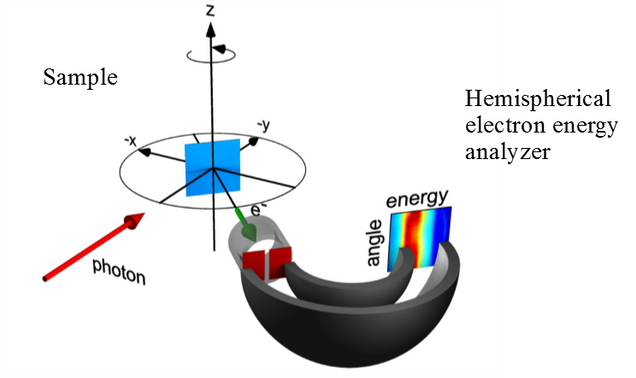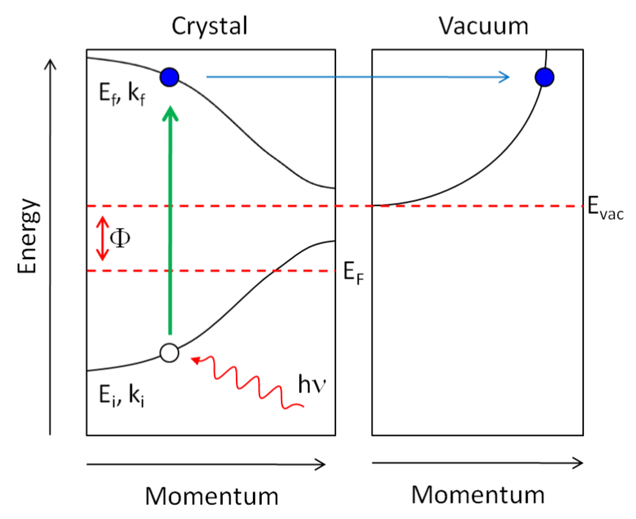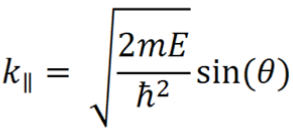# Angle Resolved Photoemission SpectroscopyBy measuring the kinetic energy (E) and momentum (k) of the electron in vacuum, the dispersion of the electron state E(k) in the solid can be determinedEF =            Fermi energy
Evac =         Vacuum level
Ei , ki=        initial energy and momentum of electron in solid
Ef , kf =      final energy and momentum of electron following excitation
E, k =         energy and momentum of free electron in vacuum after emission
Φ =             Evac – EF = work function
hν  =          incident photon energy

Energy conservation gives E(k) = hν – Φ – Ei(ki)

Momentum conservation parallel to the surface gives:Momentum is not conserved perpendicular to the surface, but for a given inner potential V0, we can write: